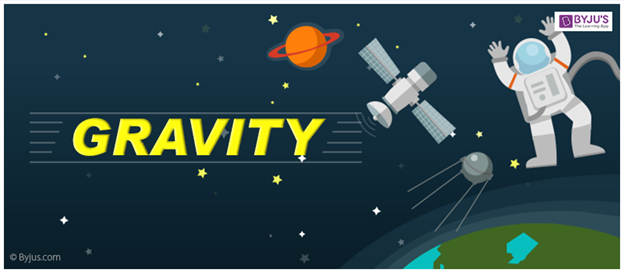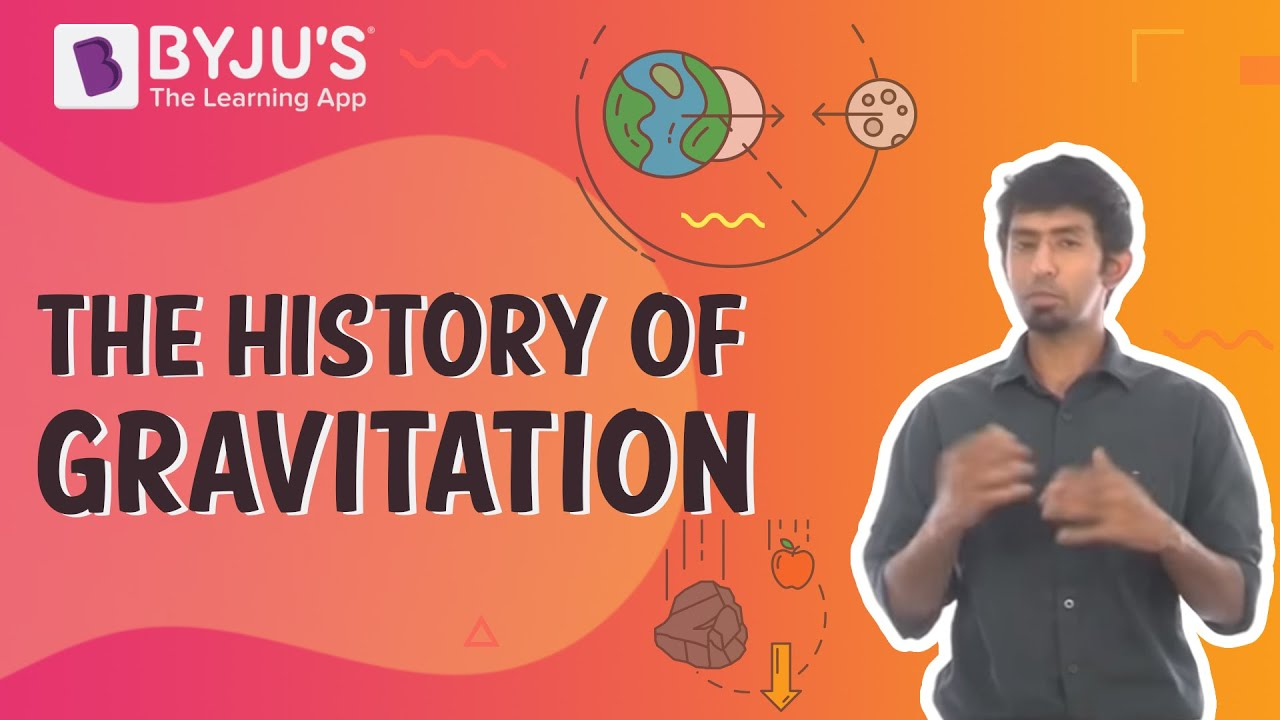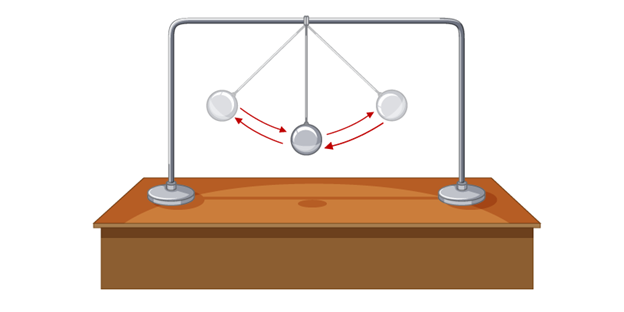# Gravity

We barely think about gravity in our daily lives, but we must realise that it defines the way we interact with the world. We grow within its constraints, and our heart, muscles, balance system all depend on it. In this article, let us understand this essential force and its effect on us.

## What is Gravity?Every object that has mass exerts a gravitational pull or force on every other mass. The strength of this pull depends on the masses of objects at play. Gravity is what keeps the planets in orbit around the sun and the moon around the Earth. Hence, we define gravity as:

Gravity is a force that attracts a body towards the centre of the earth, or towards any other physical body having mass.

### History of Gravity

• In 1687, Sir Isaac Newton first discovered gravity. While sitting under an apple tree, he noticed an apple falling down. Then he tried to figure out why the apple instead of going up or left or right, came down. In this situation, there was clearly an unknown force at play, that force is what Newton called gravity and his theory is called Newton’s law of Universal Gravitation.
• Later in the early 20th century, Albert Einstein developed a whole new idea about gravity. According to him, gravity is the warping of space and time.

### Gravity in Universe

Gravity plays a major role in sustaining life on earth. It is because of the gravitational pull between the earth and the sun the atmosphere is kept in place and provides us with the air that we need to breathe in order to survive. It also keeps us at a safe distance from the sun allowing us to enjoy the sun’s light and warmth.

### History of Gravity## Measuring Gravity

Sir Issac Newton defined the force of gravity as follows:

Every particle of matter in the universe attracts every other particle with a force that is directly proportional to the product of the masses of the particles and inversely proportional to the square of the distance between them.”

Mathematically, this translates to:

 $$F=G\frac{m_{1}m_{2}}{r^{2}}$$

Where,

• F is the force,
• $$m_1$$ and $$m_2$$ are masses of the objects interacting
• r is the distance between the center of the masses
• G is the gravitational constant (6.674×10−11 m3⋅kg−1⋅s−2)

This equation gives the magnitude of the force and since it is an attractive force it will always be directed towards the other object. From the above equation, we can come to the conclusion that anything that has mass has gravity. Objects with heavier mass have more gravity. The closer the two objects are, the heavier the gravitational pull between them. Gravity gets weaker with distance. The above formula is used to calculate the gravitational force between two objects.

## Examples of Gravity

A few examples of gravity at work are:

• The gases in the sun are held together by gravity.
• Gravity is responsible for water to rest at the bottom of a glass instead of hovering over at the top of the glass.
• The force of attraction between the earth and the moon causes tides in the ocean. This is the result of gravity.
• Gravity causes the moon to revolve around the earth.

Gravity has the same effect on all objects. If you drop an iron road and a feather, they will fall at the exact same speed. Due to the effect of air resistance on gravity, it might look like the iron rod falls at a greater speed. However, if they were dropped in the vacuum, they would fall at the exact same time. The gravity of an object depends on its size. To be more specific, the Gravity of an object depends on the mass of that object. It is undoubtedly the weakest known force in nature and thus plays no role in determining the internal properties of everyday matter.

### Relationship Between Gravity and WeightWeight is defined as the force with which a body is attracted to the earth by gravitation. It is just another word for the force of gravity Fg. Weight is a force that acts on all objects near earth. The weight of an object can be calculated by multiplying the mass of the body with the magnitude of the acceleration due to gravity (g = 9.8 m/s2).

Mathematically, it is represented as:

 Fg = mg

Where,

• Fg is the force of gravity
• m is the mass of the object
• g is the acceleration due to gravity

Many people confuse mass with weight. One has to keep in mind that mass is the measure of how much the body resists velocity, in other words, the inertia of the object. Although they are closely related to each other, they mean different things. The mass has units of kg, whereas, the weight is a force and has units of N.

• Gravity is the weakest of the four known fundamental forces of nature, yet it is the most dominant force. Even though it is the weakest force, Gravity holds together the entire solar systems and galaxies.
• The speed of free fall is consistent over the surface of the earth. This means all objects fall at the same speed. This speed on earth is calculated to be 9.8 m/s.
• The best current estimate of G is that it equals about $$6.67259\times 10^{-11}$$ newton-square meter per square kilogram.
• The force of attraction between you and the Earth is your weight.
• An object must travel 7 miles a second i.e. the earth’s escape velocity to leave Earth’s gravitational pull behind.
• The gravitational force on the moon is around 16% of that on Earth, Mars has around 38% of the Earth’s pull and Jupiter has 2.5 times the Earth’s gravity.
• Black Holes are the places in the universe that have strong gravity, that even light cannot escape from it.
• Gravity can bend light!
• According to Einstein, gravity is not a force.
• Gravity might be the weakest of the four fundamental forces, but it has an unlimited range.

Gravity is very important to us, it wouldn’t have been possible for us to live on earth if it weren’t for gravity. The sun’s gravity is what keeps the earth revolving around it, keeping us at a comfortable distance to enjoy the sun’s warmth and light. Ultimately, gravity is the glue that holds the cosmos together!

Stay tuned to BYJU’S and KEEP FALLING IN LOVE WITH LEARNING!

## Frequently Asked Questions – FAQs

### What is gravity?

Gravity is a force that attracts a body towards the centre of the earth, or towards any other physical body having mass.

### What are the examples of gravity?

The gases in the sun are held together by gravity and gravity is what causes the moon to revolve around the earth.

### Does time go faster at the top of the building than at the bottom?

Yes, time goes faster the farther away you are from the earth’s surface compared to the time on the surface of the earth. This effect is known as “gravitational time dilation”. In short, the gravitational field is nothing but the curving of space and time.

### How do spaceships create artificial gravity?

A spaceship could achieve artificial gravity by rotating about its axis. To achieve this the radius of the rotation should be large. Additionally, a ship could create artificial gravity by constantly accelerating forwards.

### What is the speed of gravity on Earth?

Gravity is measured by the acceleration it gives to free-falling objects. At Earth’s surface, the acceleration of gravity is about 9.8 meters per second.

### Does the influence of gravity extend out forever?

The farther away you get from gravitational bodies such as the sun and the Earth, its gravitational effect on you weakens but never completely goes away.